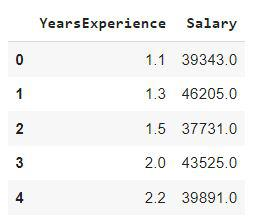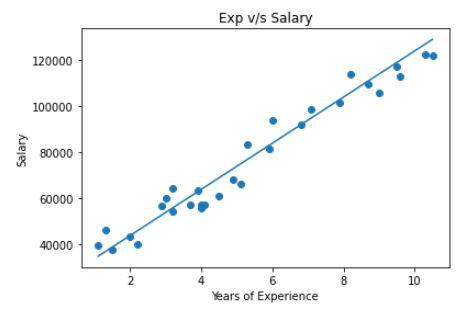Open in App
Not now

# Solving Linear Regression without using Sklearn and TensorFlow

• Last Updated : 30 Dec, 2022

In this article, we will see how can we implement a Linear Regression class on our own without using any of the sklearn or the Tensorflow API pre-implemented functions which are highly optimized for such tasks. But then why we are implementing these functions on our own? The answer to this is very simple that is because they help to clarify our concept of how to implement an idea in code.

## Importing Libraries and Dataset

Python libraries make it easy for us to handle the data and perform typical and complex tasks with a single line of code.

• Pandas – This library helps to load the data frame in a 2D array format and has multiple functions to perform analysis tasks in one go.
• Numpy – Numpy arrays are very fast and can perform large computations in a very short time.

## Python3

 `import` `numpy as np``import` `pandas as pd``import` `matplotlib.pyplot as plt`

We will be using the salary dataset here which contains only two columns having years of experience as a feature column and salary as a target column.

## Python3

 `df ``=` `pd.read_csv(``'Salary_Data.csv'``)``df.head()`

Output:First five rows of the dataset

## Python3

 `df.shape`

Output:

`(30, 2)`

Although the dataset is small it is good for demonstration purposes.

## Python3

 `X ``=` `df[``'YearsExperience'``].values.reshape(``-``1``, ``1``)``Y ``=` `df[``'Salary'``].values.reshape(``-``1``, ``1``)`

## Linear Regression

Now we will implement a LinearRegression class which will contain multiple functions which will be used for the gradient descent, updating parameters, and obtaining the trained final weights.

## Python3

 `class` `Linear_Regression():`` ` `    ``# Initiating the parameters.``    ``def` `__init__(``self``, learning_rate, no_of_itr):``        ``self``.learning_rate ``=` `learning_rate``        ``self``.no_of_itr ``=` `no_of_itr`` ` `    ``def` `fit(``self``, X, Y):`` ` `        ``# No. of training examples and no. of features.``        ``self``.m, ``self``.n ``=` `X.shape     ``# Number of rows and columns``        ``# Initiating the weight and bias``        ``self``.w ``=` `np.zeros((``self``.n, ``1``))``        ``self``.b ``=` `0``        ``self``.X ``=` `X``        ``self``.Y ``=` `Y`` ` `        ``# Implementing the gradient descent.``        ``for` `i ``in` `range``(``self``.no_of_itr):``            ``self``.update_weigths()`` ` `    ``def` `update_weigths(``self``):``        ``Y_prediction ``=` `self``.predict(``self``.X)`` ` `        ``# Calculating gradients``        ``dw ``=` `-``(``self``.X.T).dot(``self``.Y ``-` `Y_prediction)``/``self``.m`` ` `        ``db ``=` `-``np.``sum``(``self``.Y ``-` `Y_prediction)``/``self``.m`` ` `        ``# Updating weights``        ``self``.w ``=` `self``.w ``-` `self``.learning_rate ``*` `dw``        ``self``.b ``=` `self``.b ``-` `self``.learning_rate ``*` `db`` ` `    ``def` `predict(``self``, X):``        ``return` `X.dot(``self``.w) ``+` `self``.b`` ` `    ``def` `print_weights(``self``):``        ``print``(``'Weights for the respective features are :'``)``        ``print``(``self``.w)``        ``print``()`` ` `        ``print``(``'Bias value for the regression is '``, ``self``.b)`

Let’s create an object of the above class and train it for 2000 iterations with a learning rate of 0.03.

## Python3

 `model ``=` `Linear_Regression(learning_rate``=``0.03``,``                          ``no_of_itr``=``2000``)``model.fit(X_train, Y_train)`

Now let’s check the model weights which are optimized by using the gradient descent algorithm.

## Python3

 `model.print_weights()`

Output:

```Weights for the respective features are :
[[9988.54665892]]

Bias value for the regression is  23876.191228516196```

Plotting the line obtained and the along with the points will help us to visualize whether the trained weights of the Linear Regression are optimal or not.

## Python3

 `plt.scatter(df[``'YearsExperience'``], df[``'Salary'``])``plt.xlabel(``'Years of Experience'``)``plt.ylabel(``'Salary'``)``plt.title(``'Exp v/s Salary'``)`` ` `X ``=` `df[``'YearsExperience'``].values``plt.plot(X, ``9988` `*` `X ``+` `23876``)``plt.show()`

Output:Visualizing the line and the points using a scatter plot

This is quite an accurate line for the dataset we have used here. This implies that the LinearRegression model we have used here is quite accurate.

My Personal Notes arrow_drop_up